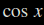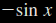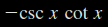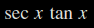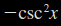# Stewart – Calculus – 3.3 – Derivatives of Trigonometric Functions$\frac{d}{\mathit{dx}}(\sin x)=?$$\frac{d}{\mathit{dx}}(\cos x)=?$$\frac{d}{\mathit{dx}}(\tan x)=?$$\frac{d}{\mathit{dx}}(\mathrm{csc} x)=?$$\frac{d}{\mathit{dx}}(\mathrm{sec} x)=?$$\frac{d}{\mathit{dx}}(\mathrm{cot} x)=?$
THE TRICK

To learn the derivatives of the 6 trig functions  you actually only have to learn 3 of them. The three to learn are sine, tangent, and secant.

 IF THEN F(X) = sin X F'(X) = cos X F(X) = tan X F'(X) = sec2 X F(X) = sec X F'(X) = sec X tan X

Cosine, Cotangent, and Cosecant.
Think of the functions as having partners:

 sine to cosine tangent to cotangent secant to cosecant

To find the derivative of the "co" functions, start with the derivatives of sine, tangent and secant, change each function in the derivative to it’s co-function partner and put a minus sign in front of the derivative.
So starting with one of the three we need to know:

If F(x) = secant X
then F'(x) = secant X tangent X

So now to get the derivative of the cosecant:
If F(X) = cosecant X
then F'(X) = -cosecant X cotangent X

So all 6 trig function derivatives look like this:

 IF: THEN: F(X) = sinX F'(X) = cosX F(X) = cosX F'(X) = – sinX F(X) = tanX F'(X) = sec2 X F(X) = cotX F'(X) = – csc2 X F(X) = secX F'(X) = sec X tan X F(X) = cscX F'(X) = -csc X cot X

Thanks to Bruce Kirkpatrick for this.